Courses

Test: Vector Analysis- 2

20 Questions MCQ Test Topicwise Question Bank for Electrical Engineering | Test: Vector Analysis- 2

Description
Attempt Test: Vector Analysis- 2 | 20 questions in 60 minutes | Mock test for Electrical Engineering (EE) preparation | Free important questions MCQ to study Topicwise Question Bank for Electrical Engineering for Electrical Engineering (EE) Exam | Download free PDF with solutions
QUESTION: 1

If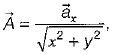then the value of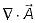at (2, 2, 0) will be

Solution:

Given,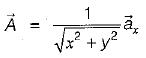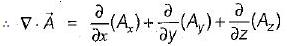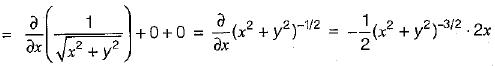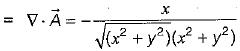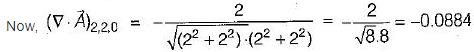QUESTION: 2

If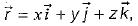then the value of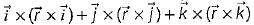is

Solution: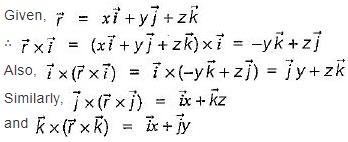Thus,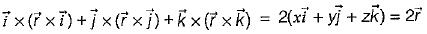QUESTION: 3

What is the value of constant b so that the vector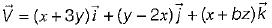is solenoidal?

Solution:

Since vector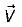is solenoidal, therefore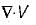= 0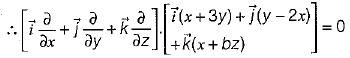or, [1 + 1 + b] = 0 or b = -2

QUESTION: 4

Match List-I with List-ll and select the correct answer using the codes given below the lists:
List-I
A. Gauss’s divergence theorem
B. Stroke’s theorem
C. The divergence
D. The curl
List-ll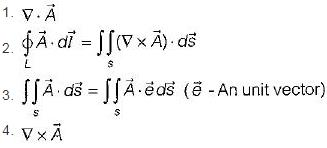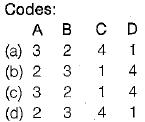Solution:
QUESTION: 5

Assertion (A): Vector differential operator is a vector quantity and it signifies that certain operations of a differentiation are to be carried out on the scalar function following it.
Reason (R): Vector differential operator posses properties similar to ordinary vectors

Solution:

Vector differential operator (‘∇’) is not a vector quantity. Hence, assertion is a false statement.

QUESTION: 6

Consider the following statements:
1. Divergence of a vector function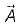at each point gives the rate per unit volume at which the physical entity is issuing from that point.
2. If a vector function ϕ represents temperature, then grad ϕ or ∇ϕ will represents rate of change of temperature with distance.
3. The curl of a vector function A gives the measure of the angular velocity at every point of the vector field.

Solution:

All the given statements are correct.

QUESTION: 7

Assertion (A): The Gauss’s divergence theorem permits us to express certain integrals by means of surface integrals.
Reason (R): Gauss’s divergence theorem states that “the surface integral of the curl of a vector field taken over any surface s is equal to the line integral of the vector field around the closed periphery (contour) of the surface.

Solution:

Reason is a statement of stroke’s theorem not that of Gauss's divergence theorem.

QUESTION: 8

If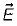is any vector field in cartesian co-ordinates system, then

Solution:

Let,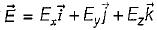be any vector field in cartesian co-ordinate system then, we can prove that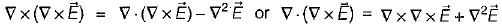Also, Div. Curl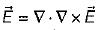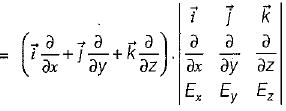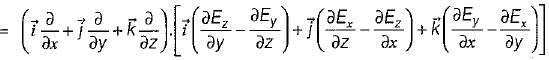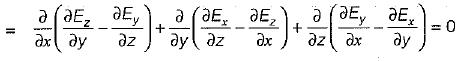QUESTION: 9

If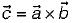and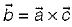, then

Solution:

Given,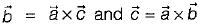∴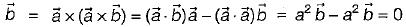Also,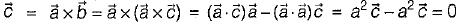QUESTION: 10

If S is any closed surface enclosing a volume V and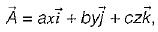then the value of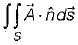(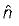is a unit vector) will be equal to

Solution: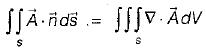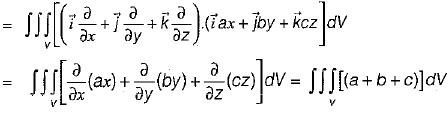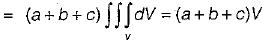QUESTION: 11

Assertion (A): The laplacian operator of a scalar function ϕ can be defined as “Gradient of the divergence of the scalar ϕ”
Reason (R): Laplacian operator may be a “scalar laplacian" or a “vector laplacian'’ depending upon whether it is operated with a scalar function or a vector, respectively.

Solution:

Assertion is not true because the laplacian operator (∇2)of a scalar function ϕ can be defined as “Divergence of the gradient of the scalar ϕ”. i.e. ∇.∇ϕ

QUESTION: 12

Match List-I (Terms) with List-II (Type) and select the correct answer using the codes given below the lists:
List-I
A. Curl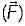= 0
B. Div= 0
C. Div grad (ϕ) = 0
D. Div div (ϕ) = 0
List-ll
1. Laplace equation
2. Irrotational
3. Solenoidal
4. Not defined
Codes:
A B C D
(a) 2 3 1 4
(b) 4 1 3 2
(c) 2 1 3 4
(d) 4 3 1 2

Solution:
QUESTION: 13

Which of the following relations are not correct?​

Solution:

(A x B)2 = A2B2 - (A·B)2

QUESTION: 14

If uF = ∇v, where u and v are scalar fields and F is a vector field, then F. curl F is equal to

Solution:

Given,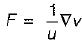∴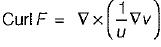or,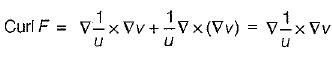Hence,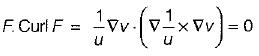QUESTION: 15

A vector field which has a vanishing divergence is called as ____________

Solution:

By the definition: A vector field whose divergence comes out to be zero or Vanishes is called as a Solenoidal Vector Field.

i.e.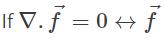is a Solenoidal Vector field.

QUESTION: 16

Which of the following statements is not true of a phasor?​

Solution:

A phasor is always a vector quantity.

QUESTION: 17

Match List-I (Physical quantities) with List-ll (Dimensions) and select the correct answer using the codes given below the lists:
List-I
A. Electric potential
B. Magnetic flux
C. Magnetic field intensity
D. Magnetic flux density
List-ll
1. MT-2I-1
2. ML2T-3I-1
3. IL-1
4. ML2T-2I-1
Codes:
A B C D
(a) 2 4 3 1
(b) 4 2 3 1
(c) 1 2 1 3
(d) 4 2 1 3

Solution:
•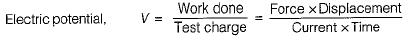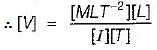• Emf induced, V = dφ/dt
or, ϕ  = v.t = Magnetic flux
∴ [ϕ] = [ML2T-3I-1[T]
= [ML2T-2I-1]
• Magnetic field intensity,
H = NI/I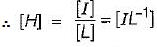Magnetic flux density B = ϕ/A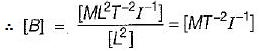QUESTION: 18

Which of the following statements is not true regarding vector algebra?

Solution:

Option (c) is not correct because cross product of two unlike vectors is a third unit vector having positive sign for normal rotation and negative for reverse rotation while cross product of two like unit vectors is zero.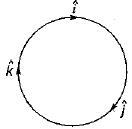QUESTION: 19

A  rigid body is rotating with an angular velocity of ω where,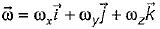and v is the line velocity. If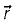is the position vector given by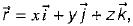then the value of curl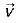will be equal to

Solution:

Taking the curl, we have: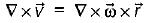(Since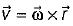)
or,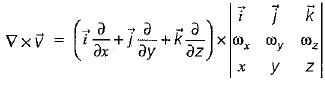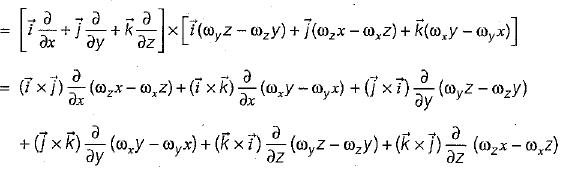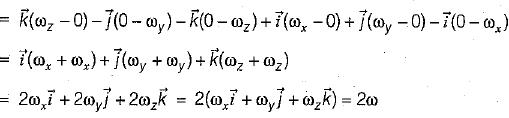∴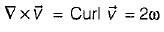QUESTION: 20

If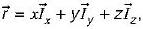then which of the following relation will hold true?

Solution:

We have: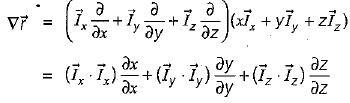= 1.1 + 1.1 + 1.1 = 1 + 1 + 1 = 3
Also,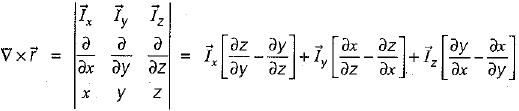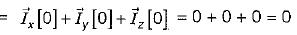Thus,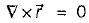Hence, both (a) and (b) will hold true.Use Code STAYHOME200 and get INR 200 additional OFF Use Coupon Code

Track your progress, build streaks, highlight & save important lessons and more!

Similar ContentRelated tests